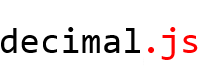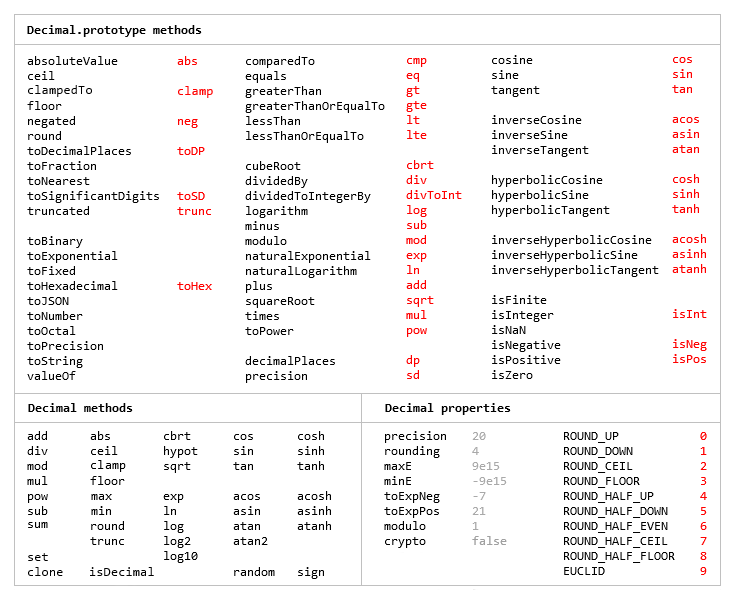{{ message }}

# MikeMcl / decimal.js

An arbitrary-precision Decimal type for JavaScript

be7d6b0

## Files

Failed to load latest commit information.
Type
Name
Commit time
May 8, 2019
Jan 10, 2017
Sep 28, 2020
Sep 28, 2020
Sep 28, 2020
Sep 28, 2020
Mar 10, 2018
Sep 28, 2020
Sep 28, 2020
Sep 28, 2020
Sep 28, 2020
Sep 28, 2020An arbitrary-precision Decimal type for JavaScript.

## Features

• Integers and floats
• Simple but full-featured API
• Replicates many of the methods of JavaScript's `Number.prototype` and `Math` objects
• Also handles hexadecimal, binary and octal values
• Faster, smaller, and perhaps easier to use than JavaScript versions of Java's BigDecimal
• No dependencies
• Wide platform compatibility: uses JavaScript 1.5 (ECMAScript 3) features only
• Comprehensive documentation and test set
• Includes a TypeScript declaration file: decimal.d.tsThe library is similar to bignumber.js, but here precision is specified in terms of significant digits rather than decimal places, and all calculations are rounded to the precision (similar to Python's decimal module) rather than just those involving division.

This library also adds the trigonometric functions, among others, and supports non-integer powers, which makes it a significantly larger library than bignumber.js and the even smaller big.js.

For a lighter version of this library without the trigonometric functions see decimal.js-light.

The library is the single JavaScript file decimal.js or ES module decimal.mjs.

Browser:

`<script src='path/to/decimal.js'></script>`
```<script type="module">
import Decimal from './path/to/decimal.mjs';
...
</script>```
`\$ npm install decimal.js`
`var Decimal = require('decimal.js');`

ES module:

```//import Decimal from 'decimal.js';
import {Decimal} from 'decimal.js';```

AMD loader libraries such as requireJS:

```require(['decimal'], function(Decimal) {
// Use Decimal here in local scope. No global Decimal.
});```

## Use

In all examples below, `var`, semicolons and `toString` calls are not shown. If a commented-out value is in quotes it means `toString` has been called on the preceding expression.

The library exports a single function object, `Decimal`, the constructor of Decimal instances.

It accepts a value of type number, string or Decimal.

```x = new Decimal(123.4567)
y = new Decimal('123456.7e-3')
z = new Decimal(x)
x.equals(y) && y.equals(z) && x.equals(z)        // true```

A value can also be in binary, hexadecimal or octal if the appropriate prefix is included.

```x = new Decimal('0xff.f')            // '255.9375'
y = new Decimal('0b10101100')        // '172'
z = x.plus(y)                        // '427.9375'

z.toBinary()                         // '0b110101011.1111'
z.toBinary(13)                       // '0b1.101010111111p+8'```

Using binary exponential notation to create a Decimal with the value of `Number.MAX_VALUE`:

`x = new Decimal('0b1.1111111111111111111111111111111111111111111111111111p+1023')`

A Decimal is immutable in the sense that it is not changed by its methods.

```0.3 - 0.1                     // 0.19999999999999998
x = new Decimal(0.3)
x.minus(0.1)                  // '0.2'
x                             // '0.3'```

The methods that return a Decimal can be chained.

```x.dividedBy(y).plus(z).times(9).floor()
x.times('1.23456780123456789e+9').plus(9876.5432321).dividedBy('4444562598.111772').ceil()```

Many method names have a shorter alias.

```x.squareRoot().dividedBy(y).toPower(3).equals(x.sqrt().div(y).pow(3))         // true
x.cmp(y.mod(z).neg()) == 1 && x.comparedTo(y.modulo(z).negated()) == 1        // true```

Like JavaScript's Number type, there are `toExponential`, `toFixed` and `toPrecision` methods,

```x = new Decimal(255.5)
x.toExponential(5)              // '2.55500e+2'
x.toFixed(5)                    // '255.50000'
x.toPrecision(5)                // '255.50'```

and almost all of the methods of JavaScript's Math object are also replicated.

```Decimal.sqrt('6.98372465832e+9823')      // '8.3568682281821340204e+4911'
Decimal.pow(2, 0.0979843)                // '1.0702770511687781839'```

There are `isNaN` and `isFinite` methods, as `NaN` and `Infinity` are valid `Decimal` values,

```x = new Decimal(NaN)                                           // 'NaN'
y = new Decimal(Infinity)                                      // 'Infinity'
x.isNaN() && !y.isNaN() && !x.isFinite() && !y.isFinite()      // true```

and a `toFraction` method with an optional maximum denominator argument

```z = new Decimal(355)
pi = z.dividedBy(113)        // '3.1415929204'
pi.toFraction()              // [ '7853982301', '2500000000' ]
pi.toFraction(1000)          // [ '355', '113' ]```

All calculations are rounded according to the number of significant digits and rounding mode specified by the `precision` and `rounding` properties of the Decimal constructor.

For advanced usage, multiple Decimal constructors can be created, each with their own independent configuration which applies to all Decimal numbers created from it.

```// Set the precision and rounding of the default Decimal constructor
Decimal.set({ precision: 5, rounding: 4 })

// Create another Decimal constructor, optionally passing in a configuration object
Decimal9 = Decimal.clone({ precision: 9, rounding: 1 })

x = new Decimal(5)
y = new Decimal9(5)

x.div(3)                           // '1.6667'
y.div(3)                           // '1.66666666'```

The value of a Decimal is stored in a floating point format in terms of its digits, exponent and sign.

```x = new Decimal(-12345.67);
x.d                            // [ 12345, 6700000 ]    digits (base 10000000)
x.e                            // 4                     exponent (base 10)
x.s                            // -1                    sign```

For further information see the API reference in the doc directory.

## Test

The library can be tested using Node.js or a browser.

The test directory contains the file test.js which runs all the tests when executed by Node, and the file test.html which runs all the tests when opened in a browser.

To run all the tests, from a command-line at the root directory using npm

`\$ npm test`

or at the test directory using Node

`\$ node test`

Each separate test module can also be executed individually, for example, at the test/modules directory

`\$ node toFraction`

## Build

For Node, if uglify-js is installed

`npm install uglify-js -g`

then

`npm run build`

will create decimal.min.js, and a source map will also be added to the doc directory.

## Licence

MIT.

See LICENCE.md

An arbitrary-precision Decimal type for JavaScript

v5.0.3 Latest
Feb 6, 2016

## Packages 0

No packages published

## Languages

You can’t perform that action at this time.